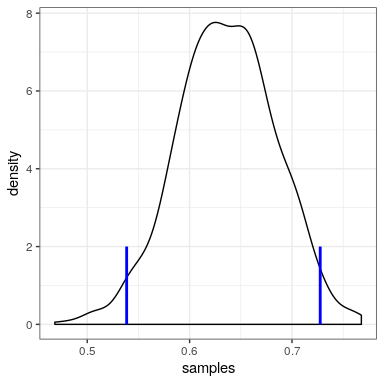# 20.8.1 Rejection sampling

We will generate samples from our posterior distribution using a simple algorithm known as rejection sampling. The idea is that we choose a random value of x (in this case $p$) and a random value of y (in this case, the posterior probability of $p$) each from a uniform distribution. We then only accept the sample if $y < f(x)$ - in this case, if the randomly selected value of y is less than the actual posterior probability of y. Figure 20.6 shows an example of a histogram of samples using rejection sampling, along with the 95% credible intervals obtained using this method.

# Compute credible intervals for example

nsamples <- 100000

# create random uniform variates for x and y
x <- runif(nsamples)
y <- runif(nsamples)

# create f(x)
fx <- dbinom(x = nResponders, size = 100, prob = x)

# accept samples where y < f(x)
accept <- which(y < fx)
accepted_samples <- x[accept]

credible_interval <- quantile(x = accepted_samples,
probs = c(0.025, 0.975))
kable(credible_interval)
x
2.5% 0.54
98% 0.73Figure 20.6: Rejection sampling example.The black line shows the density of all possible values of p(respond); the blue lines show the 2.5th and 97.5th percentiles of the distribution, which represent the 95 percent credible interval for the estimate of p(respond).